### Home > CALC > Chapter Ch3 > Lesson 3.3.2 > Problem3-110

3-110.
1. Evaluate each limit. If the limit does not exist, say so but also state if y is approaching positive or negative infinity. Homework Help ✎

1.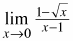2.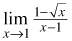3.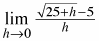4.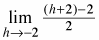5. Which problems above can be interpreted as the definition of the derivative at a point?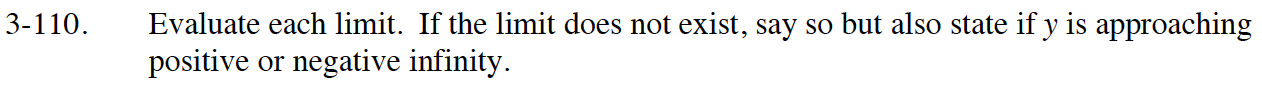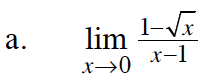Simply evaluate at x = 0.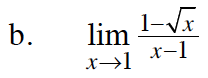$\lim_{x\rightarrow 1}\frac{1-\sqrt{x}}{x-1}\left ( \frac{1+\sqrt{x}}{1+\sqrt{x}} \right )$

$\lim_{x\rightarrow 1}\frac{1-x}{(x-1)(1+\sqrt{x})}$

$\lim_{x\rightarrow 1}\frac{-(x-1)}{(x-1)(1+\sqrt{x})}$

$\lim_{x\rightarrow 1}\frac{-1}{1+\sqrt{x}}=\underline{ \ \ \ \ \ }$

You can multiply the top and bottom by the conjugate:

You might have noticed that this is Ana's definition of the derivative:

Rewrite the given limit as:

Deconstruct the definition:

Write the derivative equation:

Evaluate at x = 1:

$\lim_{x\rightarrow a}\frac{f(a)-f(x)}{a-x}$

$-\lim_{x\rightarrow 1}\frac{\sqrt{x}-1}{x-1}$

$-f(x)=-\sqrt{x}=-x^{1/2}$

$-f'(x)=-\frac{1}{2}x^{-1/2}=-\frac{1}{2\sqrt{x}}$

a = 1

$-f'(1)=\underline{ \ \ \ \ \ \ \ }$

$\text{Either way, your answer should be }-\frac{1}{2}.$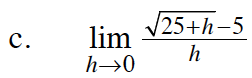This is Hana's definition of the derivative.

$\lim_{h\rightarrow 0}\frac{f(a+h)-f(a)}{h}$

$f(x)=\sqrt{x}$

a = 25

Find f '(x) and f '(25).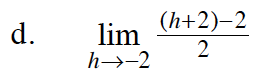Refer to the hint in part (a).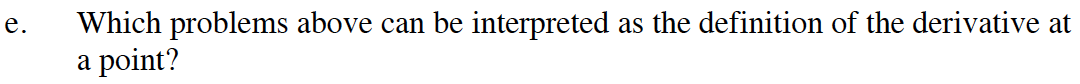Refer to the previous hints.